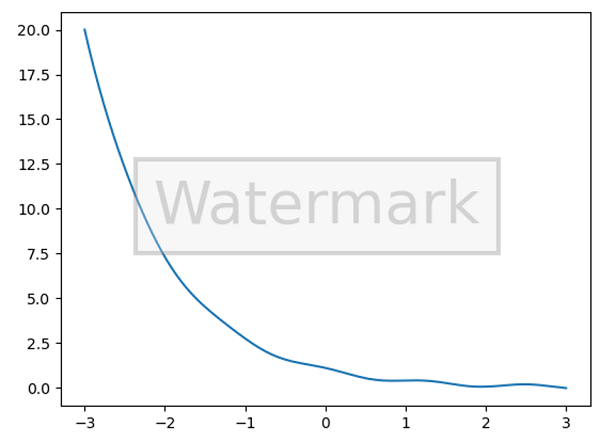# Python | Watermark in Figure using Class

Here, we are going to learn about the watermark in figure using class and its Python implementation.
Submitted by Anuj Singh, on July 19, 2020

Sometimes, we need to add watermark as a standard category of plots and therefore, we are introducing a new methodology for adding watermark in this article.## Python code for watermark in figure using class

```# Data Visualization using Python
# WaterMark in Figure

import matplotlib.pyplot as plt
from matplotlib.figure import Figure
import numpy as np

class WatermarkFigure(Figure):
def __init__(self, *args, watermark=None, **kwargs):
super().__init__(*args, **kwargs)

if watermark is not None:
bbox = dict(boxstyle='square', lw=3, ec='gray',
fc=(0.9, 0.9, .9, .5), alpha=0.3)
self.text(0.5, 0.5, watermark,
ha='center', va='center',
fontsize=40, color='gray', alpha=0.3, bbox=bbox)

x = np.linspace(-3, 3, 201)
y = np.exp(-x) + 0.1 * np.cos(5 * x)

plt.figure(FigureClass=WatermarkFigure, watermark='Watermark')
plt.plot(x, y)
```

Output:

```Output is as figure
```

Languages: » C » C++ » C++ STL » Java » Data Structure » C#.Net » Android » Kotlin » SQL
Web Technologies: » PHP » Python » JavaScript » CSS » Ajax » Node.js » Web programming/HTML
Solved programs: » C » C++ » DS » Java » C#
Aptitude que. & ans.: » C » C++ » Java » DBMS
Interview que. & ans.: » C » Embedded C » Java » SEO » HR
CS Subjects: » CS Basics » O.S. » Networks » DBMS » Embedded Systems » Cloud Computing
» Machine learning » CS Organizations » Linux » DOS
More: » Articles » Puzzles » News/Updates